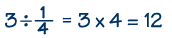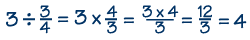Home    |    Teacher    |    Parents    |    Glossary    |    About UsDividing by fractions is just like multiplying fractions, except for one additional step.

To divide any number by a fraction:

First step: Find the reciprocal of the fraction.

Second step: Multiply the number by the reciprocal of the fraction.

Third step: Simplify the resulting fraction if possible.

Fourth step: Check your answer: Multiply the result you got by the divisor and be sure it equals the original dividend.

Note that you can only divide by non-zero fractions.

Example 1Example 2Homework Help | Pre-Algebra | FractionsEmail this page to a friendSearch·  Definitions·  Reducing fractions·  Adding and subtract-     ing fractions·  Multiplying fractions·  Dividing fractions·  Adding and subtract-     ing mixed numbers·  Multiplying     mixed numbers·  Dividing     mixed numbersFirst Glance In Depth Examples WorkoutDividing fractions